# RectangleCurve

Rectangle >

<Options>

The Rectangle command draws a closed rectangular polyline.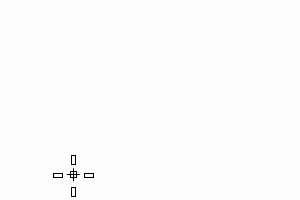#### Steps

• Pick two points for the opposite corners of a rectangle.

Or, select one of the options to draw the rectangle in a different way.

##### (Default)

Draws the rectangle using two opposite corners.##### 3Point

Draws the rectangle using two adjacent corner locations and a location on the opposite side.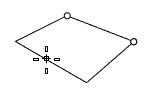##### EdgeMidpoint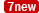Draws the rectangle from the midpoint of the first edge, an end of the edge, and a location on the opposite side.##### Vertical

Draws the rectangle perpendicular to the construction plane.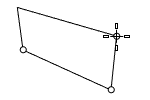##### Center

Draws the rectangle from the center point and a corner.##### AroundCurve

Draws a rectangle perpendicular to a curve.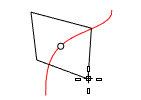#### Steps

• Select a curve and pick the center of the rectangle on the curve.
##### Rounded

Rounds the corners of the rectangle with arcs or conic sections.

#### Steps

• Pick the radius of the rounded corner.

##### Arc

Rounded corners are circular arcs.

Rounded corner